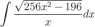# Evaluating the Integral Using Trig Substitution

• cmantzioros

#### cmantzioros

The question is to evaluate the integral in the attachment.

Using trig substition, I've reduced it to ∫ (tanz)^2 where z will be found using the triangle. I just need to integrate tangent squared which I can't seem to figure how to do. I tried using the trig identity (secx)^2 - 1 but I don't know what to do after.

#### Attachments

•integral.png
954 bytes · Views: 372
That is a good move.

Hint:
What do you get if you differentiate tan(z)?

I get (sec(z))^2)

That is to integrate tan, I said differentiate it!

Yes, sorry I realized I had made a mistake so when I differentiate tan, I get sec^2 ...

Indeed, so therefore you DO know an anti-derivative for sec^2, don't you?

Therefore, you should be able to find an anti-derivative for tan^2(z)=sec^2(z)-1

So simple... thanks a lot